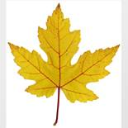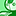# QlikView App Dev

Discussion Board for collaboration related to QlikView App Development.

cancel
Showing results for
Did you mean:Creator III

## Why is using Pick and Match functions combination thought to be faster than nested IF statements?

Hello,

I have heard from some QlikView developers that using the combination of Pick and Match functions is better than using nested IF statements to get the same output.

Can anyone describe why is using Pick and Match functions combination thought to be faster than nested IF statements please? I would like to know the background reasons1 Solution

Accepted SolutionsSpecialist III
8 RepliesSpecialist

Hello Michael,

Without commenting on performance considerations, one reason may be as the number of bucketed logical possibilities grows, from a code comprehension/maintenance perspective, Pick simplifies the syntax compared to the nested IF's.

 IF(  IF(   IF(    IF(     IF(      IF()))))))) //<~ did i miss one, is the paren depth count right?   Pick(Value, 1, 2, 3, 4, )Specialist IIIMasterMVP

In script,

I get this result

T << AUTOGENERATE(10000000) 19.995.456 lines fetched

***** if 21/10/2015 19:06:36

T2 << T 19.995.456 lines fetched

21/10/2015 19:06:47

***** pick match  21/10/2015 19:06:47

T1 << T 19.995.456 lines fetched

21/10/2015 19:07:06

with this small test script

T:

Rand() as Rand1,

Ceil(6*Rand()) as Rand2,

IterNo() as TransLineID,

RecNo() as TransID

Autogenerate 10000000

While Rand()<=0.5 or IterNo()=1;

STORE T into T.qvd (qvd);

DROP Table T;

let t=now(); trace ***** if \$(t);

T2:

TransLineID,

TransID,

if(Rand2=1, 'a',

if(Rand2=2, 'b',

if(Rand2=3, 'c',

if(Rand2=4, 'd',

if(Rand2=5, 'e',

if(Rand2=6, 'f'

))))))  as Dim2

from T.qvd (qvd);

let t=now(); trace \$(t);

DROP Table T2;

let t=now(); trace ***** pick match  \$(t);

T1:

TransLineID,

TransID,

Pick(Match(Rand2,1,2,3,4,5,6),'a','b','c','d','e','f') as Dim2

from T.qvd (qvd);

let t=now(); trace \$(t);

DROP Table T1;Contributor III

Hi ,

Can anyone formulate the below with pick and match function .

=if(PG='M'

or PG='P others'

or PG='NE'

or PG='PE'

or PG='HC'

or PG='EC'

or PG='FOOD'

or PG='BIO'

or PG='DIS

or PG='SB'

or PG='Ca'

or PG='PH

or PG='PRO'

or PG='SEYO',dual('Others',26),

if(PG='CP',dual('CPS',12),

if(PG='PC',dual('PCS',10),

if(PG='VC',dual('VCS',11),

if(PG='ALL',null(),PG)))))Specialist

I'm sure if you gave this request its own thread, someone would pitch in.Contributor III